# C++11——lambda表达式

## 1. 什么是lambda表达式？

int main() {
[](){};
return 0;
}



C++中的lambda表达式可以看作是一个匿名函数，也可以当作一个内联函数来使用。内联函数的意义在这里不展开讨论，而lambda表达式作为匿名函数在很多地方是大有用处的。

## 2. lambda表达式的结构

C++中的lambda表达式的结构如下所示：

[capture_list] (parameter_list) mutable exception -> return_type {function_body}


1.capture_list 捕获列表，列表中的局部变量可以被lambda表达式使用。
2.parameter_list 参数列表，相当于函数的参数列表。
3.mutable 用来说明捕获列表中的变量能否被修改。
4.exception 声明异常。
5.return_type 返回值类型。
6.function_body函数体。

auto L=[]{cout<<"lambda"<<endl;};


L();


[]{cout<<"lambda"<<endl;}  ();//直接调用


## 捕获列表

1.显式捕获：当需要使用到非静态局部变量的时候，需要显式地进行捕获，将该变量列入到捕获列表中。
2.隐式捕获：对于静态变量和外部变量，系统可以根据我们的使用情况推断出需要捕获的变量，因此不需要显式地将其列入捕获列表中。

## 捕获的两种模式

1.[=(identifier)] 值捕获 ；
2.[&(identifier)] 引用捕获 ；

1.[var]表示值传递方式捕捉变量var；
2.[=]表示值传递方式捕捉所有父作用域的变量（包括this，当然，“this”只能用于非静态成员函数内部）；
3.[&var]表示引用传递捕捉变量var；
4.[&]表示引用传递方式捕捉所有父作用域的变量（包括this）；
5.[this]表示值传递方式捕捉当前的this指针。

1.[=,&a,&b]表示以引用传递的方式捕捉变量a和b，以值传递方式捕捉其它所有变量;
2.[&,a,this]表示以值传递的方式捕捉变量a和this，引用传递方式捕捉其它所有变量。

3.[=,a]这里已经以值传递方式捕捉了所有变量，但是重复捕捉a了，会报错的;
4.[&,&this]这里&已经以引用传递方式捕捉了所有变量，再捕捉this也是一种重复，会报错的。

int main()
{
int id1 = 0;
int id2 = 0;
int id3 = 0;
auto f = [id1,id2,id3]()mutable
{
cout << id1++ << endl;
};
id1 = 42;
f();
f();
f();
cout << id1 << endl;
system("pause");
return 0;
}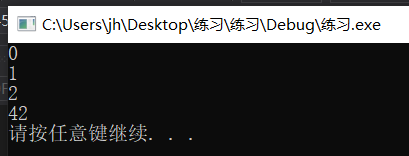int main()
{
int id1 = 0;
int id2 = 0;
int id3 = 0;
auto f = [&id1,id2,id3]() //不加mutable
{
cout << id1++ << endl;//ok
//cout<<id2++<<endl;//报错
//cout<<id3++<<endl;//报错
};
id1 = 42;
f();
f();
f();
cout << id1 << endl;
system("pause");
return 0;
}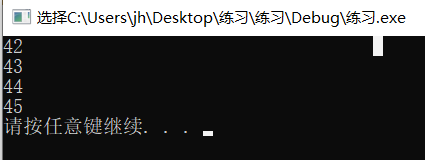## lambda表达式一个很重要的作用就是配合STL库中的各种算法，使得其结构更加简洁紧凑，功能也更加的强大。

lambda表达式是一种可调用对象。C++中可调用对象还有函数、函数指针和重载了"()"运算符的类即functor等。
1.使用functor

#include<iostream>
#include<vector>
#include<bitset>
#include<algorithm>
using namespace std;
class my_functor
{
public:
my_functor(int aa, int bb) :a(aa), b(bb) {}
bool operator()(int n)const
{
return a < n&&n < b;
}
private:
int a;
int b;
};
int main()
{
vector<int>vi = { 5,28,50,83,70,590,245,59,24 };
for (int&elem : vi)
{
cout << elem << " ";
}
cout << endl;
int x = 30, y = 100;
vi.erase(remove_if(vi.begin(), vi.end(), my_functor(x, y)), vi.end());

for (int&elem : vi)
{
cout << elem << " ";
}
cout << endl;
system("pause");

return 0;
}


2.使用lambdas表达式代替原来的functor

#include<iostream>
#include<vector>
#include<bitset>
#include<algorithm>
using namespace std;
int main()
{
vector<int>vi = { 5,28,50,83,70,590,245,59,24 };
int x = 30, y = 100;
for (int&elem : vi)
{
cout << elem << " ";
}
cout << endl;
//remove_if()将所有应该移除的元素都移动到了容器尾部并返回一个分界的迭代器
vector<int>::iterator ret_it = remove_if(vi.begin(), vi.end(), [x, y](int n) {return x < n&&n < y; });

for (int&elem : vi)
{
cout << elem << " ";
}
cout << endl;

vi.erase(ret_it, vi.end());
//或者直接合在一起写成下面的句子：
//vi.erase(remove_if(vi.begin(), vi.end(), [x, y](int n) {return x < n&&n < y; }), vi.end());

for (int&elem : vi)
{
cout << elem << " ";
}
cout << endl;
system("pause");

return 0;
}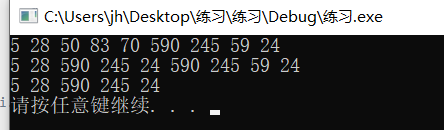int main() {
int val = 10;
vector<int> vec = { 1, 2, 3, 4, 5 };
for_each(vec.begin(), vec.end(), [=](int& elem)mutable{ elem += (--val); });
for (auto i : vec) cout << i << " ";
cout << val;
system("pause");
return 0;
}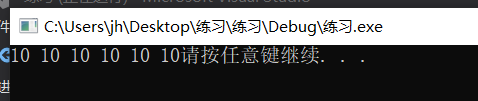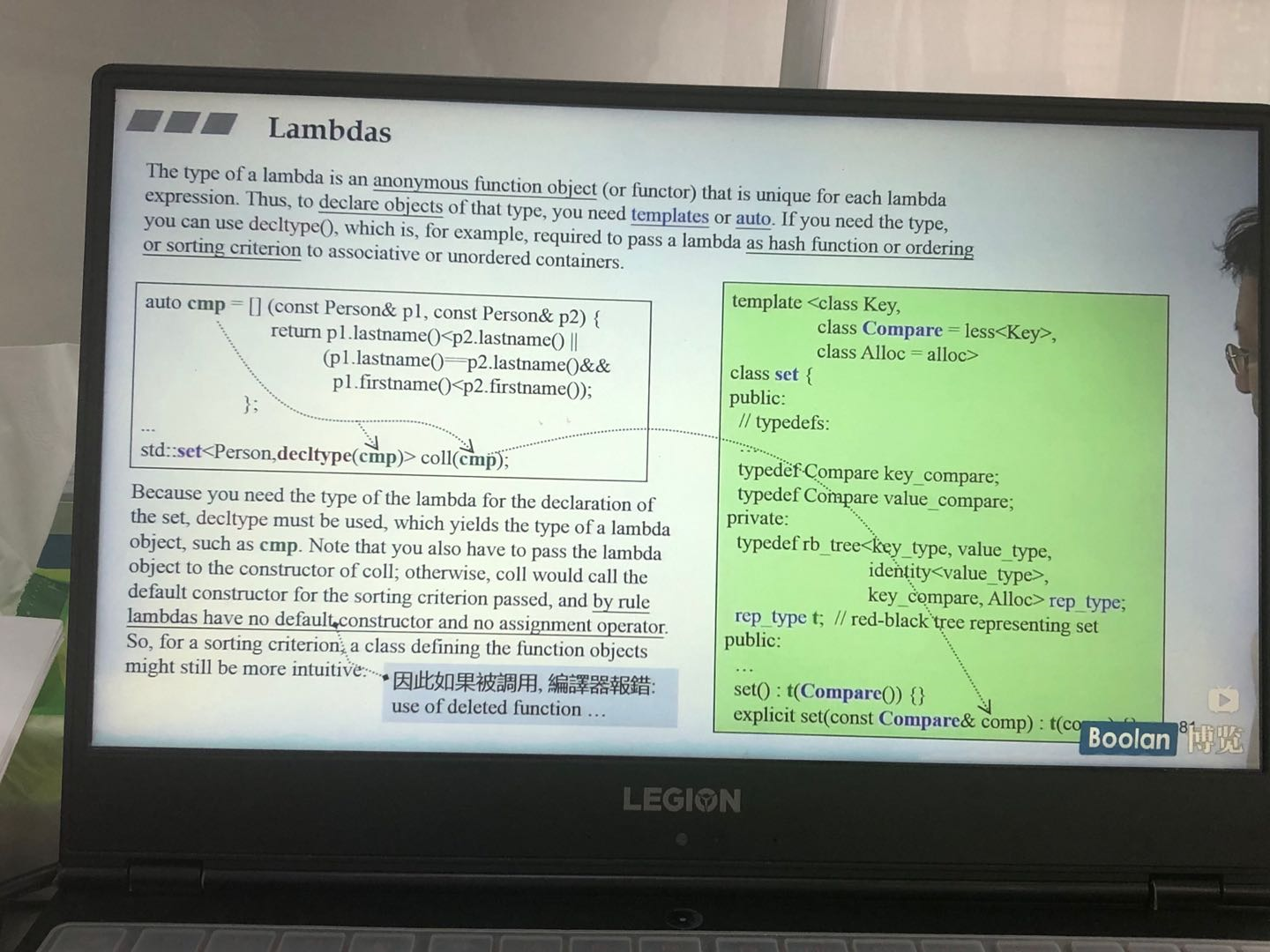1.先看functor形式：

class Customer
{
public:
string fname;
string lname;
long no;
Customer(const string _fname, const string _lname, long _no) :fname(_fname), lname(_lname), no(_no) {}
};
class mycompare
{
public:
bool operator()(const Customer&c1, const Customer&c2)const
{
return c1.no < c2.no;
}
};
int main() {
set<Customer, mycompare>myset;
myset.insert(Customer("shen","hang",1));
myset.insert(Customer("zhang", "san", 2));
/*template<class Key,
class Compare=less<Key>
class Alloc=alloc>*/
system("pause");
return 0;
}


2.再看lambda表达式形式：

class Customer
{
public:
string fname;
string lname;
long no;
Customer(const string _fname, const string _lname, long _no) :fname(_fname), lname(_lname), no(_no) {}
};

int main() {
auto mycompare = [](const Customer&c1, const Customer&c2)//lambda表达式
{
return c1.no < c2.no;
};
set<Customer, decltype(mycompare)>myset(mycompare);
myset.insert(Customer("shen","hang",1));
myset.insert(Customer("zhang", "san", 2));
/*template<class Key,
class Compare=less<Key>
class Alloc=alloc>*/
system("pause");
return 0;
}

08-14115
07-144203-1551
03-21189
04-1199
02-223310
05-275174
10-12132
09-10490
04-02907